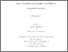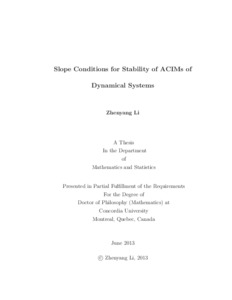Title:

# Slope Conditions for Stability of ACIMs of Dynamical Systems

Li, Zhenyang (2013) Slope Conditions for Stability of ACIMs of Dynamical Systems. PhD thesis, Concordia University.Preview
Text (application/pdf)
Li_PhD_F2013.pdf - Accepted Version
614kB

## Abstract

The family of $W-$shaped maps was introduced in 1982 by G. Keller. Based on the investigation of the properties of the maps, it was conjectured that instability of the absolutely continuous invariant measure (acim) can result only from the existence of small invariant neighbourhoods of the fixed critical point of the limiting map. We show that the conjecture is not true by constructing a family of $W-$shaped maps with acims supported on the entire interval, whose limiting dynamical behavior is described by a singular
measure. We then generalize the above result by constructing families of $W-$shaped maps $\{W_a\}$ with a turning fixed point having slope $s_1$ on one side and $-s_2$ on the other. Each such $W_a$ map has an acim $\mu_a$. Depending on whether $\frac{1}{s_1}+\frac{1}{s_2}$ is larger, equal, or smaller than 1, we show that the limit of $\mu_a$ is a singular measure, a combination of singular and absolutely continuous measure or an acim, respectively. We also consider $W$-shaped maps satisfying $\frac 1{s_1}+\frac 1{s_2}=1$ and show that the eigenvalue $1$ of the associated Perron-Frobenius operator is not stable, which in turn implies the instability of the isolated spectrum. Motivated by the above results, we introduce the harmonic average of slopes condition, with which we obtain new explicit constants for the upper and lower bounds of the invariant probability density function associated with the map, as well as a bound for the speed of convergence to the density. Moreover, we prove stability results using Rychlik's Theorem together with the harmonic average of slopes condition for piecewise expanding maps.

Divisions: Concordia University > Faculty of Arts and Science > Mathematics and Statistics Thesis (PhD) Li, Zhenyang Concordia University Ph. D. Mathematics 18 June 2013 Gora, Pawel and Boyarsky, Abraham Dynamical systems, Absolutely continuous invariant measures, Slope conditions, W-shaped maps 977389 ZHENYANG LI 13 Jan 2014 15:22 18 Jan 2018 17:44
All items in Spectrum are protected by copyright, with all rights reserved. The use of items is governed by Spectrum's terms of access.

Repository Staff Only: item control pageResearch related to the current document (at the CORE website)
Back to top